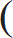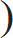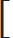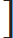# Problems on Percentage - Aptitude test, questions, shortcuts, solved example videos

Video on Problems on Percentage - shortcuts, tips and tricks

## Percentage

Points to Remember
 1) Y % is expressed as Y 100

 2) To find percent of x =x × 100% y y

Quick Tips and Tricks

1. Prices of Goods

(1) If the price of goods increases by R %, then the reduction in consumption so as not to increase the expenditure can be calculated using the formula:R × 100% (100 + R)

(2) If the price of goods decreases by R %, then the increase in consumption so as not to decrease the expenditure can be calculated using the formula:R × 100% (100 – R)

2. Numerical on Population: Population of a city at present is P and it increases at the rate of R% per annum.
 (1) To find population after n years = P1 + Rn 100

 -------- (After n years population increases, thus1 + Ris used) 100

(2) To find population n years ago =
P1 + Rn 100

3. Numerical on Depreciation: Present value of machine is M, If it depreciates at the rate of R% per annum.
 (1) To find value of machine after n years = P1 – Rn 100

 -------- (After n years the value of machine decreases, thus1 – Ris used) 100

(2) To find the value of machine n years ago =
P1 – Rn 100

Question Variety

Generally 5 types of questions are asked from this chapter. Understanding these concepts will help in solving the problems related to this chapter.

Type 1: Numerical on numbers

Q 1. The difference between two numbers is 1550. If 8 % of one number is 10 % of the other number, then find the two numbers

a. 4973, 6523
b. 5450, 7000
c. 6200, 7750
d. 6500, 4950
View solution

Correct Option: (c)

Let two numbers be x and y.
It is given that, 8 % of x = 10 % of y
Therefore,

 x = 10 y = 5 y 8 4
Difference between two numbers (x – y)= 1550
Substituting the value of x, we get
 5 – y = 1550 4
 y = 1550 4
y=1550×4=6200
 x = 5 × 6200 = 7750 4

The two numbers whose difference is 1550 are 6200 and 7750.

Q 2. Two numbers P and Q are such that, the sum of 2 % of P and Sum of 2 % of Q is two-third of the sum of 2 % of P and 6 % of Q. Find the ratio of P and Q.

a. 2 : 5
b. 3 : 1
c. 1 : 4
d. 5 : 1
View solution

Correct Option: (b)

The sum of 2 % of P and Sum of 2% of Q is two-third of the sum of 2 % of P and 6 % of Q.
This sentence means that,

 2% of P + 2% of Q = 2 (2% of P + 6% of Q) 3
 2 P + 2 Q = 22 P + 6 Q100 100 3 100 100
 1 P + 1 Q =1 P + 1 Q50 50 75 251 – 1P =1 – 150 75 25 50
 1 P = 1 Q 150 50
 P = 150 = 3 Q 50 1

Alternate method
 2 P + 2 Q = 22 P + 6 Q100 100 3 100 100
Simply eliminate 100 from both the sides, we get
3(2P + 2Q) = 2(2P + 6Q)
6P + 6Q = 4P + 12Q
6P – 4P =12Q – 6Q
2P = 6Q
 P = 6 = 3 Q 2 1

Q 3. 50 % of a number is 18 less than two-third of that number. Find the number.

a. 123
b. 119
c. 115
d. 108
View solution

Correct Option: (d)

Let the number be x.
It is given that, 50 % of a number is 18, less than two-third of that number. This means that,

 2 x – (50% of x) = 18 3
 2 x – 50 x = 18 3 100
50x=5400
x=108
The number is 108

Q 4. When 35 is subtracted from a number; it reduces to its 80 %. Find the four-fifth of that number.

a. 140
b. 125
c. 137
d. 129
View solution

Correct Option: (a)

We are given, 35 when subtracted from a number, reduces to its 80 %.
Therefore,
Let the number be x.

 x – 35 = 80 x 100
Solving, we get the value of x
 x – 80 x = 35 100
 x = 35 × 100 x = 175 20
Now, we are asked to find the four-fifth of that number i.e x
 4 x = 4 × 175 = 140 5 5
Hence, the number is 140.

Type 2: Numerical on Depreciation

Q 5. The value of lathe machine depreciates at the rate of 10 % per annum. If the cost of machine at present is Rs. 160,000, then what will be its worth after 2 years?

a. Rs. 122,365
b. Rs. 153,680
c. Rs. 129,600
d. Rs. 119,900
View solution

Correct Option: (c)

Hint: Present value of machine is M. If the cost depreciates at the rate of R% per annum, then

 The value of machine after n years = P1 – Rn 100
In this numerical, we are given the present cost of the machine i.e 160,000 and the cost decreases by 10 % per annum. We have to find the cost of this machine after 2 years.
We can solve this numerical in a minute, if we know the trick used to solve such numerical related to depreciation of cost.

Given:
Present amount = Rs. 1,60,000
Rate of depreciation = 10 %
Substituting the given values, we get
 The value of machine after n years = P1 – Rn 100
 = 1,60,000 ×1 – 102 100
= Rs. 129,600
After 2 years, the cost of machine = Rs. 129,600

Q 6. The value of Xerox machine depreciates at the rate of 10 % per annum. If the cost of machine at present is Rs. 75,000 then what was the value of machine before 2 years?
a. Rs. 90,000
b. Rs. 92,600
c. Rs. 93,800
d. Rs. 95,000
View solution

Correct Option: (b)

Hint: Present value of machine is M. If the cost depreciates at the rate of R% per annum, then

The value of machine n years ago =
P1 – Rn 100

Given:
Cost of Xerox machine at present = Rs. 75,000
Rate of depreciation = 10 %
Substituting the given values, we get
The value of machine n years ago =
P1 – Rn 100
=
750001 – 102 100
=Rs.92592.60
Therefore, the value of machine before 2 years = Rs. 92592.60
But this value is not available in the given options. Hence, select the nearby value to the amount of Rs. 92592.60. Among the listed options option (b) is the correct answer.

Type 3: Numerical on Population

Q 7. The current birth rate per thousand is 30, whereas corresponding death rate is 10 per thousand. Find the net growth rate in terms of population increase in percent.

a. 1.5 %
b. 2 %
c. 2.5 %
d. 3 %
View solution

Correct Option: (b)

We are given that,
1) Current birth rate per thousand is 30
2) Corresponding death rate is 10 per thousand

Hence, net growth on 1000 = Current birth rate - death rate
= 30 – 10 =20
We are asked to find, net growth rate in terms of population increase in percent (which means net growth on 100)

 Net worth on 100 = Net worth on 1000 × 100 1000
 Net worth on 100 = 20 × 100 = 2% 1000

Q 8. The total population of a city is 6500.The number of males and females increases by 5 % and 10 % respectively and consequently the population becomes 7000. Find the number of males in the village.

a. 4000
b. 3000
c. 3500
d. 2950
View solution

Correct Option: (b)

We are given that,
1) Total population of city = 6500
2) Increase in male and female population = 5 % & 10% respectively.
3) Final population of city = 7000
Hence,
Let’s assume that number of males = x
Number of female = 6500 – x
Therefore, after increase in 5 % male and 10 % female, the population becomes 7000
5 % male +10 % female = Difference between new and original population

 5 x + 10 (6500 – x) = 7000 – 6500 100 100
5x+65000-10x=50000
5x= 15000
x=3000
Number of males = 3000
Number of females = 3500

Q 9. The present population of a country is 10 crores. If it rises to 17.28 crores during next 3 years, then find uniform rate of growth in population.

a. 20 %
b. 30 %
c. 40 %
d. 60 %
View solution

Correct Option: (a)

Hint:

 To find population after n years = P1 + Rn 100

Here, we know
1) Present population of country = 10 crores
2) After 3 years, population of country = 17.28 crores
To find: Rate of growth R%
After 3 years, the population is 17.28 crores. Therefore,
 P1 + Rn = 17.28 100
 101 + R3 = 17.28 1001 + R3 = 17.28 = 1728 =123 100 10 1000 101 + R= 12 100 101 + R= 1 + 2 100 10
 R = 2 100 10
R=20%
Rate of growth in population = 20 %

Q 10. The population of different trees in a field increased by 10 % in first year, increased by 8 % in second year and decreased by 10 % in third year. If at present the number of trees is 26730, then find the number of tress in the beginning.

a. 30000
b. 25000
c. 27000
d. 27865
View solution

Correct Option: (b)

Hint:

To find population n years ago =
P1 + Rn 100

We are given, number of trees increased in
1) First year = increased by 10 %
2) Second year = increased by 8 %
3) Third year = decreased by 10 %

Therefore, to find number of trees in the beginning use the trick.
Population n years ago =
267301 + 101 + 81 + 10100 100 100
 = 26730 × 10 × 25 × 10 11 27 9
= 25000

Type 4: Numerical on Prices of Goods

Q 11. The price of diesel increases by 50 %. Find by how much percent a truck owner must reduce his consumption in order to maintain the same budget?

a. 11.11 %
b. 22.22 %
c. 33.33 %
d. 44.44 %
View solution

Correct Option: (c)

Hint: If the price of goods increases by R %, then the reduction in consumption so as not to increase the expenditure can be calculated using the formula:R × 100% (100 + R)
 =50 × 100% (100 + 50)
= 33.33 %

The truck owner must reduce its consumption in order to maintain the same budget by 33.33 %

Q 12. The price of rice falls by 15 %. By what percentage a person can increase the consumption of rice so that his overall budget does not change?

a. 10.74 %
b. 17.64 %
c. 20.46 %
d. 21.90 %
View solution

Correct Option: (b)

Hint: If the price of goods decreases by R %, then the increase in consumption so as not to decrease the expenditure can be calculated using the formula:R × 100% (100 – R)

Using this trick, we can easily solve such type of numerical.
The price of rice falls by 15 %, therefore substituting this value, we get15 × 100% = 17.64 % (100 – 15)
Therefore, the person can increase his consumption by 17.64 %

Type 5: Numericals based on Marks of students

Q 13. In an examination, P scored 30 % marks and failed by 15 marks. Q scored 40 % marks and obtained 35 marks more than those required to pass. Find the pass percentage.

a. 30 %
b. 33 %
c. 35 %
d. 40 %
View solution

Correct Option: (b)

We have to calculate the pass percentage.
In case of P: He scores 30 % out of total marks, but fails by 15 marks. Hence, the simple equation formed is (30 % of x) + 15
In case of Q: He scores 40 % out of total marks, but gets 35 marks more than required to pass. Hence, the simple equation formed is (40 % of x) – 35

1) First calculate total marks.
Let total marks be x.
(30 % of x) + 15 = (40 % of x) – 35

 30 × x + 15 = 40 × x – 35 100 100
 35 + 15 = 40 × x – 30 × x 100 100
 50 = 1 x 10
x=500
Total marks = 500

2) As we know the total marks, we can calculate the passing.
 Passing Marks = 30 × x + 15 100
 Passing Marks = 30 × 500 + 15 = 165 100
Therefore,
 Passing Percentage = 165 × 100 = 33% 500

Required pass percentage = 33 %

Q 14. In a science examination, the average obtained by entire class was 80 marks. If 10 % of students scored 92 marks and 20 % of students scored 90 marks, then what was the average of remaining students?

a. 65.32
b. 70.56
c. 75.43
d. 77.96
View solution

Correct Option: (c)

Here, we do not know the number of students in the class. So let the number of students be 100 and the required average is y.
1) 10 % of students scored 92 marks
2) 20 % of students scored 90 marks
3) Therefore, from 100 students, the remaining students are 70
4) Average obtained by 100 students = 80 marks

Considering the given parameters, form the equation.
(10 x 92) + (20 x 90) + (70 x y) = (100 x 80)

70 y = 8000 – (1800 + 920)
y = 75.43
The average of remaining students = 75.43

Q 15. A student attempts x number of questions. He answers 15 correctly out of first 20 questions and of the remaining questions, he answers 1/3 correctly. If all questions have same credit and the student gets 50 % marks, then find the value of x.

a. 30
b. 35
c. 45
d. 50
View solution

Correct Option: (d)

Given:
1) Student attempts x questions.
2) Out of 20 questions he answers 15 correctly and of (x – 20) questions he answered 1/3 correctly.
3) The student gets 50 % marks.
Therefore,

 15 + 1 (x – 20) = 50% of x 3
 15 + 1 (x – 20) = 50 × x 3 100
 15 + 1 (x – 20) = x 3 2
90+2 (x-20)=3x
Solving this equation, we get
x=50
Hence, the number of question attempted by the students = 50

Profit and Loss - Aptitude test, questions, shortcuts, solved example videos
Profit and Loss - Quantitative aptitude tutorial with easy tricks, tips, short cuts explaining the concepts. Online aptitude preparation material with practice question bank, examples, solutions and explanations. Video lectures to prepare quantitative aptitude for placement tests and competitive exams like MBA, Bank exams, RBI, IBPS, SSC, SBI, RRB, Railway, LIC, MAT. Very useful for freshers, engineers, software developers taking entrance exams. Learn and take practice tests!
Average - Aptitude test, questions, shortcuts, solved example videos
Average - Quantitative aptitude tutorial with easy tricks, tips, short cuts explaining the concepts. Online aptitude preparation material with practice question bank, examples, solutions and explanations. Video lectures to prepare quantitative aptitude for placement tests and competitive exams like MBA, Bank exams, RBI, IBPS, SSC, SBI, RRB, Railway, LIC, MAT. Very useful for freshers, engineers, software developers taking entrance exams. Learn and take practice tests!
Problem on Ages - Aptitude test, questions, shortcuts, solved example videos
Problems on Ages - Quantitative aptitude tutorial with easy tricks, tips, short cuts explaining the concepts. Online aptitude preparation material with practice question bank, examples, solutions and explanations. Video lectures to prepare quantitative aptitude for placement tests and competitive exams like MBA, Bank exams, RBI, IBPS, SSC, SBI, RRB, Railway, LIC, MAT. Very useful for freshers, engineers, software developers taking entrance exams. Learn and take practice tests!